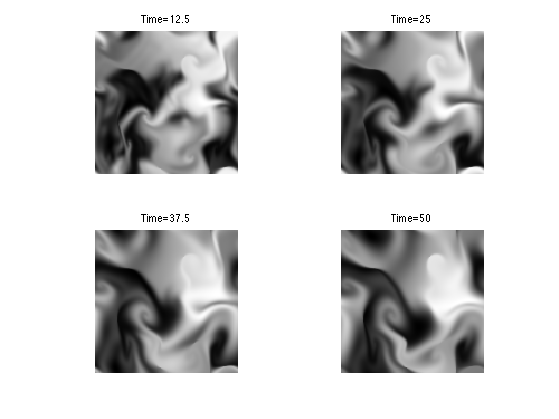$\newcommand{\NN}{\mathbb{N}} \newcommand{\CC}{\mathbb{C}} \newcommand{\GG}{\mathbb{G}} \newcommand{\LL}{\mathbb{L}} \newcommand{\PP}{\mathbb{P}} \newcommand{\QQ}{\mathbb{Q}} \newcommand{\RR}{\mathbb{R}} \newcommand{\VV}{\mathbb{V}} \newcommand{\ZZ}{\mathbb{Z}} \newcommand{\FF}{\mathbb{F}} \newcommand{\KK}{\mathbb{K}} \newcommand{\UU}{\mathbb{U}} \newcommand{\EE}{\mathbb{E}} \newcommand{\Aa}{\mathcal{A}} \newcommand{\Bb}{\mathcal{B}} \newcommand{\Cc}{\mathcal{C}} \newcommand{\Dd}{\mathcal{D}} \newcommand{\Ee}{\mathcal{E}} \newcommand{\Ff}{\mathcal{F}} \newcommand{\Gg}{\mathcal{G}} \newcommand{\Hh}{\mathcal{H}} \newcommand{\Ii}{\mathcal{I}} \newcommand{\Jj}{\mathcal{J}} \newcommand{\Kk}{\mathcal{K}} \newcommand{\Ll}{\mathcal{L}} \newcommand{\Mm}{\mathcal{M}} \newcommand{\Nn}{\mathcal{N}} \newcommand{\Oo}{\mathcal{O}} \newcommand{\Pp}{\mathcal{P}} \newcommand{\Qq}{\mathcal{Q}} \newcommand{\Rr}{\mathcal{R}} \newcommand{\Ss}{\mathcal{S}} \newcommand{\Tt}{\mathcal{T}} \newcommand{\Uu}{\mathcal{U}} \newcommand{\Vv}{\mathcal{V}} \newcommand{\Ww}{\mathcal{W}} \newcommand{\Xx}{\mathcal{X}} \newcommand{\Yy}{\mathcal{Y}} \newcommand{\Zz}{\mathcal{Z}} \newcommand{\al}{\alpha} \newcommand{\la}{\lambda} \newcommand{\ga}{\gamma} \newcommand{\Ga}{\Gamma} \newcommand{\La}{\Lambda} \newcommand{\Si}{\Sigma} \newcommand{\si}{\sigma} \newcommand{\be}{\beta} \newcommand{\de}{\delta} \newcommand{\De}{\Delta} \renewcommand{\phi}{\varphi} \renewcommand{\th}{\theta} \newcommand{\om}{\omega} \newcommand{\Om}{\Omega} \renewcommand{\epsilon}{\varepsilon} \newcommand{\Calpha}{\mathrm{C}^\al} \newcommand{\Cbeta}{\mathrm{C}^\be} \newcommand{\Cal}{\text{C}^\al} \newcommand{\Cdeux}{\text{C}^{2}} \newcommand{\Cun}{\text{C}^{1}} \newcommand{\Calt}{\text{C}^{#1}} \newcommand{\lun}{\ell^1} \newcommand{\ldeux}{\ell^2} \newcommand{\linf}{\ell^\infty} \newcommand{\ldeuxj}{{\ldeux_j}} \newcommand{\Lun}{\text{\upshape L}^1} \newcommand{\Ldeux}{\text{\upshape L}^2} \newcommand{\Lp}{\text{\upshape L}^p} \newcommand{\Lq}{\text{\upshape L}^q} \newcommand{\Linf}{\text{\upshape L}^\infty} \newcommand{\lzero}{\ell^0} \newcommand{\lp}{\ell^p} \renewcommand{\d}{\ins{d}} \newcommand{\Grad}{\text{Grad}} \newcommand{\grad}{\text{grad}} \renewcommand{\div}{\text{div}} \newcommand{\diag}{\text{diag}} \newcommand{\pd}{ \frac{ \partial #1}{\partial #2} } \newcommand{\pdd}{ \frac{ \partial^2 #1}{\partial #2^2} } \newcommand{\dotp}{\langle #1,\,#2\rangle} \newcommand{\norm}{|\!| #1 |\!|} \newcommand{\normi}{\norm{#1}_{\infty}} \newcommand{\normu}{\norm{#1}_{1}} \newcommand{\normz}{\norm{#1}_{0}} \newcommand{\abs}{\vert #1 \vert} \newcommand{\argmin}{\text{argmin}} \newcommand{\argmax}{\text{argmax}} \newcommand{\uargmin}{\underset{#1}{\argmin}\;} \newcommand{\uargmax}{\underset{#1}{\argmax}\;} \newcommand{\umin}{\underset{#1}{\min}\;} \newcommand{\umax}{\underset{#1}{\max}\;} \newcommand{\pa}{\left( #1 \right)} \newcommand{\choice}{ \left\{ \begin{array}{l} #1 \end{array} \right. } \newcommand{\enscond}{ \left\{ #1 \;:\; #2 \right\} } \newcommand{\qandq}{ \quad \text{and} \quad } \newcommand{\qqandqq}{ \qquad \text{and} \qquad } \newcommand{\qifq}{ \quad \text{if} \quad } \newcommand{\qqifqq}{ \qquad \text{if} \qquad } \newcommand{\qwhereq}{ \quad \text{where} \quad } \newcommand{\qqwhereqq}{ \qquad \text{where} \qquad } \newcommand{\qwithq}{ \quad \text{with} \quad } \newcommand{\qqwithqq}{ \qquad \text{with} \qquad } \newcommand{\qforq}{ \quad \text{for} \quad } \newcommand{\qqforqq}{ \qquad \text{for} \qquad } \newcommand{\qqsinceqq}{ \qquad \text{since} \qquad } \newcommand{\qsinceq}{ \quad \text{since} \quad } \newcommand{\qarrq}{\quad\Longrightarrow\quad} \newcommand{\qqarrqq}{\quad\Longrightarrow\quad} \newcommand{\qiffq}{\quad\Longleftrightarrow\quad} \newcommand{\qqiffqq}{\qquad\Longleftrightarrow\qquad} \newcommand{\qsubjq}{ \quad \text{subject to} \quad } \newcommand{\qqsubjqq}{ \qquad \text{subject to} \qquad }$

Fluid Dynamics

# Fluid Dynamics

This numerical tour explores fluid dynamics for image generation.

## Installing toolboxes and setting up the path.

You need to download the following files: signal toolbox and general toolbox.

You need to unzip these toolboxes in your working directory, so that you have toolbox_signal and toolbox_general in your directory.

For Scilab user: you must replace the Matlab comment '%' by its Scilab counterpart '//'.

Recommandation: You should create a text file named for instance numericaltour.sce (in Scilab) or numericaltour.m (in Matlab) to write all the Scilab/Matlab command you want to execute. Then, simply run exec('numericaltour.sce'); (in Scilab) or numericaltour; (in Matlab) to run the commands.

Execute this line only if you are using Matlab.

getd = @(p)path(p,path); % scilab users must *not* execute this


Then you can add the toolboxes to the path.

getd('toolbox_signal/');
getd('toolbox_general/');


## Velocity Flow Field

A velocity flow is simply a 2-D vector field $$V = (V_i)_{i=1}^N \in \RR^{n \times n \times 2}$$ where $$V_i \in \RR^2$$ is one of the $$N=n \times n$$ vectors at a position indexed by $$i$$.

It can be generated as a realization of Gaussian process. The blurring creates correlations in the flow.

n = 128;
options.bound = 'per';
V = perform_blurring(randn(n,n,2), 40, options);


Subsampling display operator.

myplot = @(V)plot_vf( V(1:6:n,1:6:n, :) );


We can display the vector field using arrow.

clf;
myplot(V);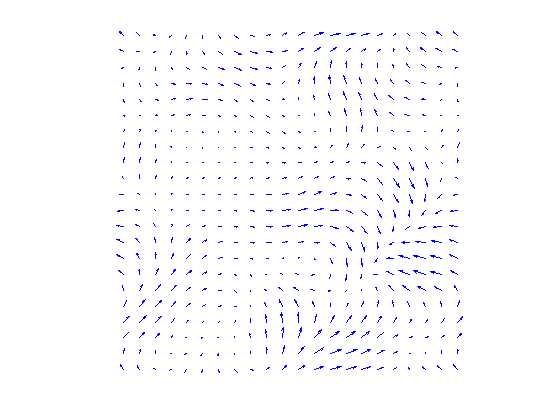We can renormalize the flow, which enhance the singularities. It defines $$\tilde V$$ as $$\tilde V_i = V_i/\norm{V_i}$$.

normalize = @(V)V ./ repmat( max(1e-9,sqrt(sum3(V.^2, 3))) , [1 1 2]);


Display.

clf;
myplot(normalize(V));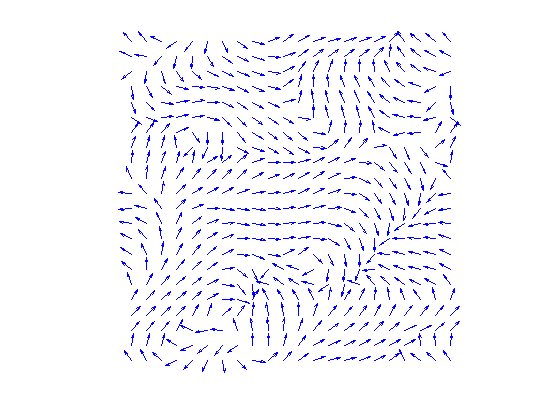## Incompressible Flows

An incompressible flow as vanishing divergence. The set of vector incompressible flow defines a sub-space of $$\RR^{n \times n \times 2}$$ $\Ii = \enscond{V}{ \text{div}(V)=0 } \qwhereq \text{div}(V) = \pd{V}{x_1} + \pd{V}{x_2} \in \RR^{n \times n}.$ Here $$\pd{}{x_s}$$ for $$s=1,2$$ are finite differences approximation of the horizontal and vertical derivative operators (we suppose here periodic boundary conditions).

The orthogonal projection $$U = \text{Proj}_{\Ii}(V)$$ on $$\Ii$$ is computed by solving a Poisson equation $U = V-\nabla A \qwhereq \Delta A = \text{div}(V).$

This is especially simple for periodic boundary conditions since $$A$$ can be compute over the Fourier domain as $\forall \om \neq 0, \quad \hat A(\om) = \frac{\hat Y(\om)}{\mu(\om)} \qwhereq Y = \text{div}(V) \qandq \mu(\om_1,\om_2) = -4 \sin(\om_1 \pi / n)^2 -4 \sin(\om_2 \pi / n)^2$ and $$\hat A(0)=0$$.

Compute the kernel $$\mu(\om)$$.

[Y,X] = meshgrid(0:n-1,0:n-1);
mu = sin(X*pi()/n).^2; mu = -4*( mu+mu' );
mu(1) = 1;


Computation of $$A$$.

A = @(V)real( ifft2( fft2( div(V, options) ) ./ mu ) );


Projection on incompressible flows.

ProjI = @(V)V - grad(A(V), options);


Display $$U=\text{Proj}_{\Ii}(V)$$.

U = ProjI(V);
clf;
myplot(U);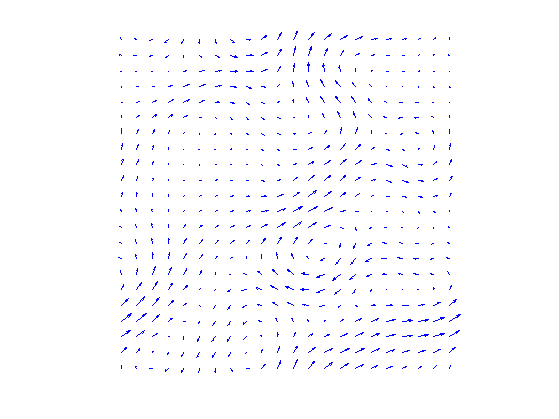Display $$W=U-V$$ the irrotational component of $$V$$.

clf;
myplot(V-U);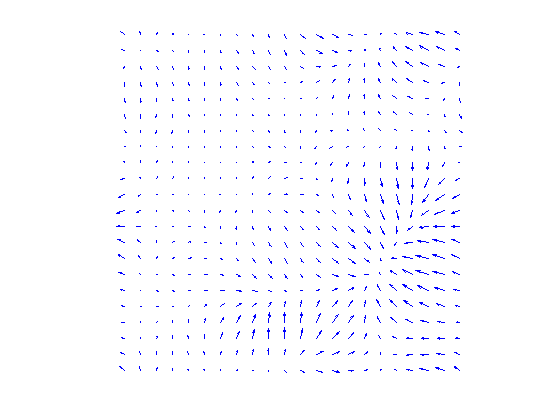Note that the decomposition $$V=U+W$$ is called the Hoge decomposition of the vector field.

## Image Advection Along the Flow

A flow defines a warping operator that transport the content of an image along the streaming of the flow.

We load an image $$f$$.

name = 'lena';
f = crop( load_image(name, 2*n), n);


Given some vector field $$U$$, the warping operator $$f_1 = \Ww_U(f)$$ along the flow is define $f_1(x) = f(x+U(x))$ i.e. it advects the values of $$f$$ by the vector field $$U$$ to obtain the values of $$f_1$$.

We define $$U$$ as a scaled normalized incompressible flow.

U = normalize(ProjI(V));


Helper function: enforce periodicity.

periodic = @(P)cat(3, mod(P(:,:,1)-1,n)+1, mod(P(:,:,2)-1,n)+1 );


Helper function: extend an image by 1 pixel to avoid boundary problems.

extend1 = @(f)[f f(:,1)];
extend = @(f)extend1(extend1(f)')';


Helper function: bilinear interpolation on a grid.

myinterp = @(P1,f1,Pi)interp2( P1(:,:,2), P1(:,:,1),f1, Pi(:,:,2), Pi(:,:,1) );


First we compute the initial and warped grids.

[Y,X] = meshgrid(1:n,1:n);
P = cat(3, X,Y);
[Y1,X1] = meshgrid(1:n+1,1:n+1);
P1 = cat(3, X1,Y1);


Defines the warping operator $$\Ww_U$$.

W = @(f,U)myinterp( P1, extend(f), periodic( P - U ) );


Display a warped image $$\Ww_{\rho U}(f)$$ for some scaling $$\rho$$.

rho = 2;
clf;
imageplot(W(f,rho*U));Exercice 1: (check the solution) Display $$\Ww_{\rho U}(f)$$ for various values of $$\rho$$.

exo1;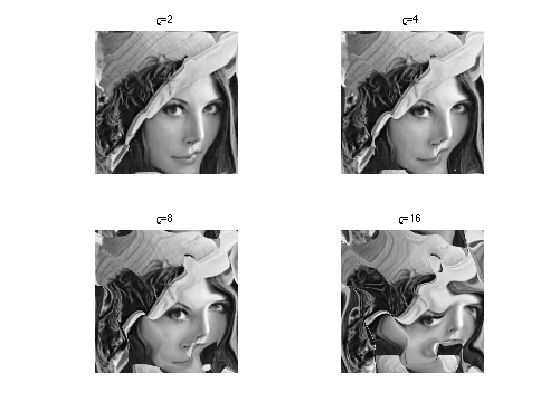Exercice 2: (check the solution) Define an iterative scheme via: $f^{(\ell+1)} = \Ww_{\rho U}(f^{(\ell)}).$ Display the result $$f^{(\ell)}$$, which corresponds approximately to solving an advection equation at time $$t=\ell \rho$$.

exo2;## Fluid Dynamics

Fluid dynamics solves the incompressible Navier-Stokes equations to evolve in time the vector field.

We discribe here a simple algorithm introduced in:

J. Stam, Stable Fluids, SIGGRAPH'99, 1999, p. 121-128.

It proposes a semi-implicit scheme for the resolution of the Navier Stockes equations for the movement of incompressible fluids $\pd{V}{t} = \text{Proj}_{\Ii}\pa{ -(V \cdot \nabla) V + \nu \Delta V + W }.$ Here $$\nu \geq 0$$ is the viscosity of the fluid, $$W$$ is a source term, $$\Delta$$ is the Laplacian, and $$-(V \cdot \nabla) V$$ is the non-linear self-advection, where we have used the short-hand notation $$V \cdot \nabla$$ for the derivative operator along a flow $$V$$: $(V \cdot \nabla)U = ( V_1 \pd{U_1}{x_1} + V_2 \pd{U_1}{x_2}, V_1 \pd{U_2}{x_1} + V_2 \pd{U_2}{x_2} ).$

In order to visualize the flow, we also advect and diffuse along the flow a density $$g$$ of particules, which is a scalar field. Once $$V$$ has been computed, it follows a linear PDE $\pd{g}{t} = -(V \cdot \nabla) g + \mu \Delta g + h$ with some initial condition at time $$t=0$$, where $$h$$ is a source for the density.

In practice, we solve this PDE in parallel to the PDE for $$V$$.

In the following, we use $$W=0$$ and $$h=0$$ (no sources).

Set the viscosity $$\nu$$ for the velocity field.

nu = 1/10;


We use a larger viscosity $$\mu$$ for the evolution of the density of particules.

mu = 2*nu;


Extend the warping operator $$\Ww_U$$ to work with vector fields as input. This will apply $$\Ww_U$$ on each channel of the vector field (X and Y coordinates).

Wt = @(V,U)cat(3, W(V(:,:,1),U), W(V(:,:,2),U) );


We discretize the PDE's using some time step $$\tau$$.

tau = .5;


The algorithm computes $$V^{(\ell)}$$ at iteration $$\ell$$ which is an approximation of the PDE solution at time $$\ell \tau$$. It is computed itertatively as $\tilde V^{(\ell)} = \Ww_{\tau V^{(\ell)}}( V^{(\ell)} ) \qandq V^{(\ell+1)} = \text{Proj}_{\Ii}\pa{ \tilde V^{(\ell)} + \tau\nu\Delta \tilde V^{(\ell)} + \tau W }$

It computes in parallel the evolution of the density as $\tilde g^{(\ell)} = \Ww_{\tau V^{(\ell)}}( g^{(\ell)} ) \qandq g^{(\ell+1)} = \tilde g^{(\ell)} + \tau\nu\Delta \tilde g^{(\ell)} + \tau h$

Set the initial field $$V=V^{(0)}$$ at time $$t=0$$.

V = normalize(ProjI(V));


Set the initial density $$g=g^{(0)}$$ at time $$t=0$$.

g = f;


The first step is to advect the vector field $$V$$ and $$g$$ along the flow $$V$$ itself. This corresponds to an implict discretization of the term $$-(V \cdot \nabla) V$$.

g = W (g,tau*U);
V = Wt(V,tau*U);


We implement the Laplacian using finite difference.

s1 = [2:n 1]; s2 = [n 1:n-1];
Delta = @(g)1/4 *( g(s1,:,:) + g(s2,:,:) + g(:,s1,:) + g(:,s2,:) ) - g;


The second step is to diffuse the vector field and the density.

V = V + tau*nu*Delta(V);
g = g + tau*mu*Delta(g);


The last step is to ensure incompressibility of $$V$$ by projecting on $$\Ii$$.

V = ProjI(V);


Exercice 3: (check the solution) Compute the fluid dynamic by iterating these steps.

exo3;